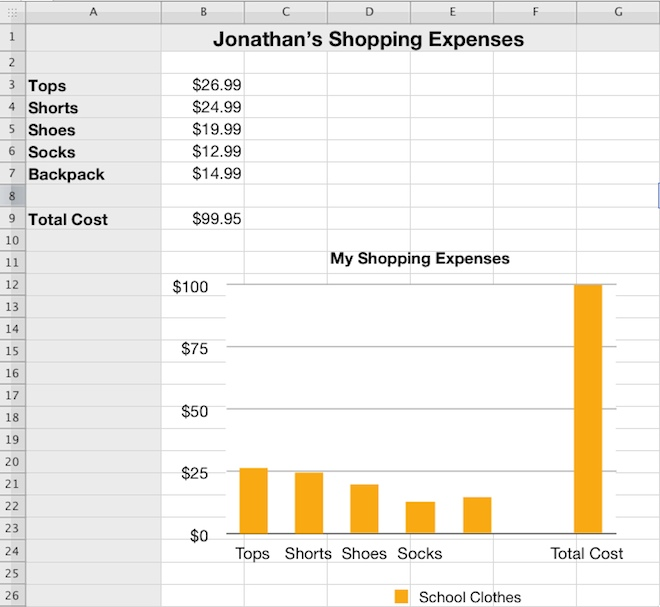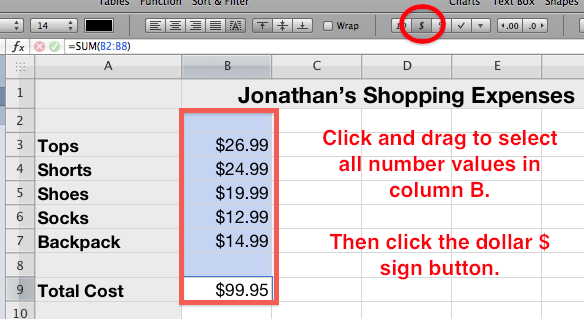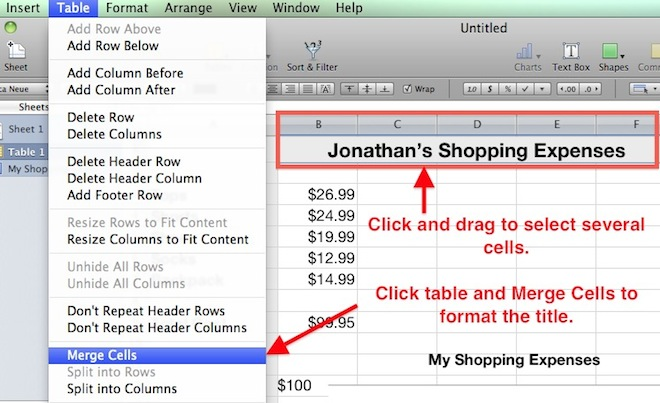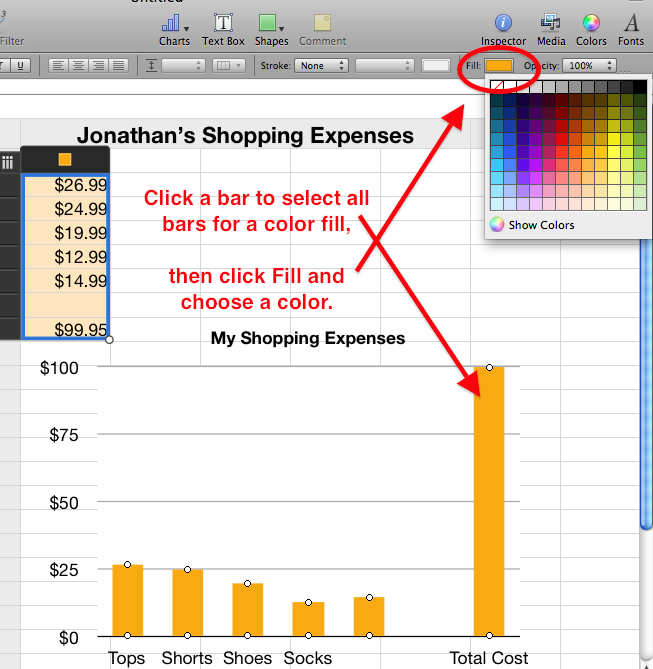# iWork Numbers - Expense Chart

## Students Create a Simple Expense Spreadsheet and Column Chart

Students learn the following skills:

1) Students enter all data into the spreadsheet.
2) Students use the sum function to calculate the total expenses.
3) Students format all data to a larger font size and format the numbers with dollar signs.

4) Students select all data and create a basic bar chart.

5) Students merge the table cells to format the title (Jonathan's Shopping Expenses) across the table.

### Instructions:

Students enter 5 items and limit their total expenditures to \$100.00 or less.

Students enter numerical values for each item and then use the SUM function to automatically calculate the total.

Students can revise their costs and let the spreadsheet formula automatically re-calculate the total until it is less than or equal to \$100.00.

## Finished Expense Spreadsheet and Chart Example:## Use the Sum Function to Calculate Total Expenses## Format the Numbers with Dollar Signs## Merge Cells to Format Title## Choose a Color Fill for the Chart Bars